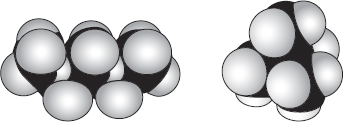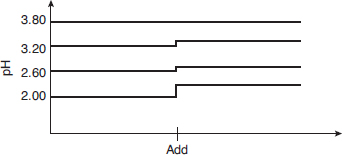# AP Chemistry Practice Test 26

### Test Information12 questions18 minutes

1.n-pentane (C5H12 or CH3CH2CH2CH2CH3) and 2,2-dimethylpropane (C5H12 or (CH3)4C), shown above as space-filling models, each have the same number of carbon and hydrogen atoms but the atoms are arranged differently. n-pentane boils at 36.1 °C, and 2,2-dimethylpropane boils at 9.5 °C. Which statement best explains these data?

`Questions 2-5 refer to the following information.Questions refer to the cell diagrams and their corresponding E°cell values for the three galvanic cells listed in the data table below.Galvanic CellCell DiagramE°cell1Zn | Zn2+(1.0 M) || Cu2+(1.0 M) | Cu1.102Cd | Cd2+(1.0 M) || Cu2+(1.0 M) | Cu0.743Zn | Zn2+(1.0 M) || Cd2+(1.0 M) | Cd?The chemical reaction occurring in the second galvanic cell isCd + Cu2+ → Cd2+ + Cu`

2. What is the standard cell potential for galvanic cell 3 depicted in the table above?

3. What is the chemical reaction under study in galvanic cell 3?

4. If the concentration of Cd2+ is changed from 1.0 M to 0.1 M in the galvanic cells above, what will happen to the observed cell voltages in galvanic cells 2 and 3?

5. Rank Cd, Cu, and Zn from the metal that is easiest to oxidize to the metal that is the most difficult to oxidize.

6. Magnesium has an atomic radius of 160 pm and a first ionization energy of 737 kJ.

Based on periodic trends and the data given above, what are the most probable values for the ionization energy and atomic radius of sodium?

7. Iron is slowly oxidized by the permanganate in the reaction

5Fe2+ + 8H+ + MnO4- → 5Fe3+ + Mn2+ + 4H2O

The decrease in absorbance of a dilute solution of permanganate is followed using a spectrometer set to the appropriate wavelength in the visible region of the spectrum. The table below provides the data collected in this experiment.

 Time (h) Absorbance (A) ln A 1/A 0 0.75 -0.29 1.3 1 0.38 -0.97 2.6 2 0.19 -1.7 5.3 3 0.095 -2.4 11

Which of the following is the best interpretation of the data?

8. 0.0025 mol of a weak, monoprotic acid is dissolved in 0.250 L of distilled water. The pH was then measured as 4.26. What is the pKa of this weak acid?

`Questions 9-12 refer to the following information.Four different acid solutions of 0.0100 M are prepared, and their pH values are recorded on a laptop computer. One of the solutions contains more than just an acid. At the point designated as "add," all four solutions are diluted with an equal volume of water. The bottom line represents solution 1, and the top line represents solution 4.`

9. Which of the four acids is best described as a strong acid?

10. Using the data in the graph, which solution seems to be a buffer?

11. Which solution contains only an acid and the acid is the weakest of all?

12. Which acid(s) will require the most 0.0050 M NaOH to neutralize 25.0 mL of a 0.010 M solution of the acid?